Localizing and excluding quantum informa-
tion; or, how to share a quantum secret in
spacetime
Patrick Hayden
1
and Alex May
2
1
Stanford University
2
The University of British Columbia
October 15, 2019
When can quantum information be localized to each of a collection of
spacetime regions, while also excluded from another collection of regions?
in which a quantum system must be localized to a collection of authorized
regions while also being excluded from a set of unauthorized regions. This
task is a spacetime analogue of quantum secret sharing, with authorized and
unauthorized regions replacing authorized and unauthorized sets of parties.
Our analysis yields the ﬁrst quantum secret sharing scheme for arbitrary ac-
cess structures for which the number of qubits required scales polynomially
with the number of authorized sets. We also study a second related task
called state-assembly, in which shares of a quantum system are requested at
sets of spacetime points. We fully characterize the conditions under which
both the localize-exclude and state-assembly tasks can be achieved, and give
explicit protocols. Finally, we propose a cryptographic application of these
tasks which we call party-independent transfer.
Patrick Hayden: phayden@stanford.edu
Alex May: may@phas.ubc.ca
Accepted in Quantum 2019-07-02, click title to verify 1
arXiv:1806.04154v4 [quant-ph] 14 Oct 2019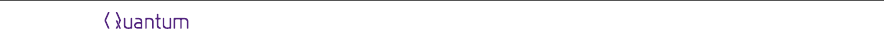Contents
1 Introduction 2
2 Localizing and excluding quantum information 4
2.1 Localizing quantum information to many regions . . . . . . . . . . . . . 4
2.2 Localizing and excluding quantum information . . . . . . . . . . . . . . 10
3 State-assembly 19
3.1 State-assembly with authorized regions . . . . . . . . . . . . . . . . . . 19
3.2 State-assembly with authorized and unauthorized regions . . . . . . . . 23
4 An application: party-independent transfer 24
5 Discussion 29
6 Acknowledgements 31
A Summoning, state-assembly and localization 31
B Many-call single-return summoning 35
1 Introduction
The study of the interplay between quantum theory and relativity has recently begun
a new chapter with the consideration of quantum information tasks in a Minkowski
space background [1, 2, 3]. For instance, the study of information causality  and of
causal operators  has given further insight into ties between information processing
and relativity. Along with other results in this area [6, 7, 8, 9], these can be placed into
the general framework of quantum tasks in Minkowski space .
One task of particular interest is summoning, deﬁned by Kent , where the associ-
ated no-summoning theorem is a statement of no-cloning appropriate to the spacetime
setting. We have also argued that a generalization of the summoning task  provides
an operational framework within which to study how quantum information can move
through spacetime. The importance of having such a framework is highlighted by recent
subtle questions concerning spacetime structure and the no-cloning principle in the con-
text of black holes [12, 13]. Understanding how a quantum system may be delocalized
in Minkowski space should be a useful step towards understanding such fundamental
puzzles.
The study of quantum tasks in Minkowski space has been given a second motivation
with the discovery of cryptographic protocols that exploit the properties of either or
both of quantum mechanics and special relativity. Bit-commitment is a well-known
example [14, 15]; other examples include coin ﬂipping , key distribution (where sig-
nalling constraints enter into some security proofs [17, 18]), and two spacetime analogues
Accepted in Quantum 2019-07-02, click title to verify 2A
1
A
2
s
U
1
x
t
Figure 1: An example of a localize-exclude task. A single copy of an unknown quantum system is
initially localized near the spacetime point s, and needs to be localized to within regions A
1
and
A
2
, while avoiding region U
1
. Theorem 8 shows that this is possible to do.
of oblivious transfer dubbed location-oblivious transfer  and spacetime-constrained
oblivious transfer .
In quantum secret sharing, a central result of quantum cryptography, a quantum
system is distributed among many parties such that only certain subsets of parties may
collectively use their shares to reconstruct the system. Other subsets of parties are
required to not be able to learn any information about the secret from their shares. In
the context of quantum tasks in Minkowski space, where the movement of information
in spacetime is central, and in the context of relativistic quantum cryptography, it is
natural to consider a spacetime generalization of quantum secret sharing.
To do this we replace the notions of authorized and unauthorized sets of parties with
authorized and unauthorized spacetime regions. We deﬁne the localize-exclude task,
where the goal is to move a quantum system through spacetime in such a way that
it is localized to each of the authorized regions and excluded from the unauthorized
ones. Figure 1 gives a simple example. In theorem 8, we ﬁnd necessary and suﬃcient
conditions for completing the localize-exclude task. To argue that the localize-exclude
task is a natural spacetime generalization of secret sharing, we show in the main text
that there is a simple construction that embeds any quantum secret sharing scheme
as a localize-exclude task, and that the conditions of this theorem reduce to those for
quantum secret sharing in that case.
In the summoning task one party, Bob, puts in requests for the quantum system at
certain spacetime points, asking that the system be returned at one of another set of
points. The localize-exclude task removes this structure, but adds a notion of unautho-
Accepted in Quantum 2019-07-02, click title to verify 3rized region. It is interesting to also consider a task in the request-return setting, but
which includes unauthorized regions. In this state-assembly task, we consider many
parties Bob
i
who may each request a share of the quantum system at an associated
spacetime region D
i
. Alice should respond to the collection of requests given by the
Bobs in a careful way: she should hand over a collection of shares suﬃcient to construct
a single copy of the system when the collection of requests is authorized, and she should
not reveal any information about the system when that collection is unauthorized. The
conditions for Alice to complete this task are the same as for localize-exclude in the
case of causally separated regions, but diﬀer when non-trivial causal structures are con-
sidered. In theorem 13 below we precisely characterize the conditions under which this
task can be completed, and describe an explicit protocol for completing it when it is
possible.
Together the state-assembly and localize-exclude tasks provide a rich set of scenarios
to consider. We suggest party-independent transfer as a potential cryptographic appli-
cation of this framework, a task where two other parties wish to receive information
from Alice and want the information they receive to be both private and independent
of their identity. We propose a protocol for completing this task which is built on the
state-assembly task. Establishing the security of this protocol we leave to future work.
The layout of this paper is as follows. Section 2 gives the necessary deﬁnitions to
study localization to arbitrary spacetime regions and proves theorem 8, which charac-
terizes the localize-exclude task. We discuss the relation between localize-exclude and
quantum secret sharing in the same section. In section 3 we discuss state-assembly and
give its characterization. In section 4 we study the party-independent transfer task.
Two appendices are included which clarify the relationship of this work to earlier work
on summoning. The ﬁrst shows that state-assembly is equivalent to a certain summon-
interpreting summoning tasks in terms of the localization of information.
2 Localizing and excluding quantum information
2.1 Localizing quantum information to many regions
As a ﬁrst step towards characterizing the localize-exclude task we discuss the problem
of localizing quantum information to a collection of spacetime regions, leaving excluded
regions to the next section. To do this we consider the following setting. Alice holds the
A subsystem of a pure state |Ψi
RA
, with A recorded into a collection of classical and
quantum systems held within secure laboratories not accessible to her adversary, Bob
1
.
We would like to ask where system A is. For instance, Alice might have recorded A into
an error-correcting code and distributed the shares of this code to various laboratories.
1
Alice and Bob are both agencies, who have many agents that may be distributed to many diﬀerent
laboratories.
Accepted in Quantum 2019-07-02, click title to verify 4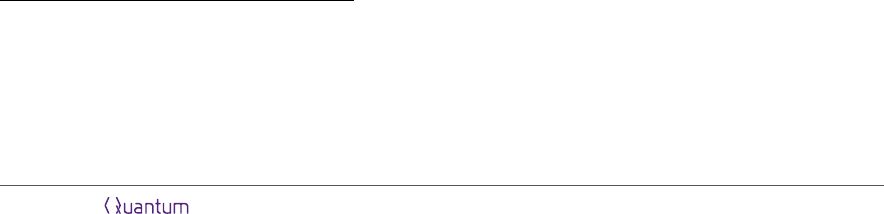Further, she might be constantly rerouting these shares between labs, so that shares
are held only at certain labs between speciﬁed times.
We can ask where the subsystem is in spacetime by temporarily relaxing the security
of Alice’s labs we give Bob access to some collection of Alice’s labs for certain time
intervals. If by accessing these labs Bob is able to prepare the A system (potentially
making use of later data processing), we say that system A was localized to the collection
of labs and intervals of time Bob accessed. More generally, we can abstract away from
the language of labs and time intervals and give a more general deﬁnition.
Deﬁnition 1 Suppose one party, Alice, holds system A of a quantum state |Ψi
AR
.
Then we say the subsystem A is localized to a spacetime region Σ if a second party,
Bob, for whom the state is initially unknown is able to prepare the A system by collecting
quantum and classical systems from within Σ, and then applying later data processing.
Conversely, if Bob is unable to learn anything about A we say the system is excluded
from Σ. Note that the later data processing referred to in the deﬁnition may occur
outside of the region Σ. Further, a system may be neither localized nor excluded from
a region if partial information about the system is available there.
To be more precise we should specify how it is veriﬁed that Bob holds the A system
after he has accessed Σ. One natural possibility is to introduce a third party, call him
Charlie, who plays the role of a referee. We have Charlie hold both the purifying system
R of |Ψi
AR
as well as a classical description |Ψi
AR
. To verify Bob holds the system then,
we have Bob pass the A system to Charlie, who performs a projective measurement
of the AR system in a basis that includes |Ψi
AR
. If Alice can pass Charlie’s test with
certainty, we declare that Alice localized the system to Σ. Of course, Alice will also pass
this test with some probability so long as Charlie’s ﬁnal state has non-trivial overlap
with |Ψi
AR
2
.
It is interesting to compare this notion of localizing a quantum system to a spacetime
region to a notion of spacetime localization based on the summoning task . Perhaps
the key distinction is that, in the deﬁnition given here, information processing may
occur outside the spacetime region in order to prepare the system. This point carries
with it certain subtleties that are taken up in appendix A and the discussion. The key
advantage of deﬁnition 1 however is its applicability to regions of arbitrary shape.
One strategy for hiding a quantum system from Bob would be for Alice to send A
into a region Σ, while also sending various decoys A
d1
, A
d2
, ... so that Bob, though he
may collect all of the systems A, A
d1
, A
d2
, ... is left unsure as to which system to hand
to Charlie. This reveals a ﬁner point to deﬁnition 1: the system Bob is searching for
may enter Σ, but if appropriate classical instructions do not also enter Σ (in this case
2
In the context of the localize-exclude tasks we consider later, we may be interested in whether or
not a quantum system can be localized to two or more regions with ﬁxed relative positions, rather than
if a system can be localized to one particular spacetime region. In this case, and when the spacetime
is suitably translation invariant, one can consider repeating the task many times (sequentially or in
parallel). In this scenario Charlie could determine with what probability Alice is able to complete the
Accepted in Quantum 2019-07-02, click title to verify 5a label denoting which system actually holds A), then deﬁnition 1 says the system is
not localized there. To avoid confusion around this point we will always have Alice, at
some early time, reveal the classical instructions that constitute her protocol to Bob.
The only information Alice will not broadcast is a classical string k (as well as the
quantum system itself). As we will see, protocols where Alice holds only a secret key
k and reveals all other details to Bob are suﬃcient to complete any physically possible
localize-exclude task, so this restriction on Alice amounts to a useful simpliﬁcation of
notation and language.
In the protocols we construct Alice will encode her quantum system into an error-
correcting code that corrects erasure errors, and then apply a quantum one-time pad
to each of the shares in the quantum code. Alice does not broadcast the classical
strings used in the one-time pads; taken together these constitute her secret key k.
However, she does reveal her procedure for putting A into an error-correcting code and
applying the one-time pad, and reveals the spacetime trajectories of each share in the
code. Within this context, Bob reconstructs A by accessing a region Σ whenever a
correctable subset of shares in the error-correcting code along with their corresponding
classical keys from the the one-time pad pass through Σ.
Deﬁnition 1 speciﬁes what is meant by a quantum system being localized to a single
spacetime region. To extend this to multiple regions, we deﬁne the localize task as
follows.
Deﬁnition 2 A localize task is a task involving two agencies, Alice and Bob, speciﬁed
by a tuple {A, s, {A
1
, ..., A
n
}}, consisting of:
A quantum system A. In general A may be a subsystem of some overall pure state
|Ψi
AR
. The state on AR is unknown to both Alice and Bob.
A start point s, at which Alice initially holds system A
A collection of spacetime regions {A
1
, ..., A
n
}, which we call the authorized regions
Alice successfully completes the task if Bob is able to prepare system A after he accesses
any one of the A
i
.
If Alice is able to successfully complete the localize task with regions {A
1
, ..., A
n
} we
say she has localized the system to each of those regions. The authorized regions may
be of arbitrary shape and may overlap.
To analyze this task it is useful to introduce some language. We give the following
deﬁnition which speciﬁes a relation between pairs of spacetime regions.
Deﬁnition 3 Two spacetime regions Σ
i
and Σ
j
are said to be causally connected if
there is a point q
i
in Σ
i
and q
j
in Σ
j
such that there is a causal curve from q
i
to q
j
, or
from q
j
to q
i
.
We illustrate this deﬁnition in ﬁgure 2a. If two regions are not causally connected we
say they are causally disjoint. In the context of the localize-exclude task discussed in
the next section we will also need one further deﬁnition relating to spacetime geometry.
Accepted in Quantum 2019-07-02, click title to verify 6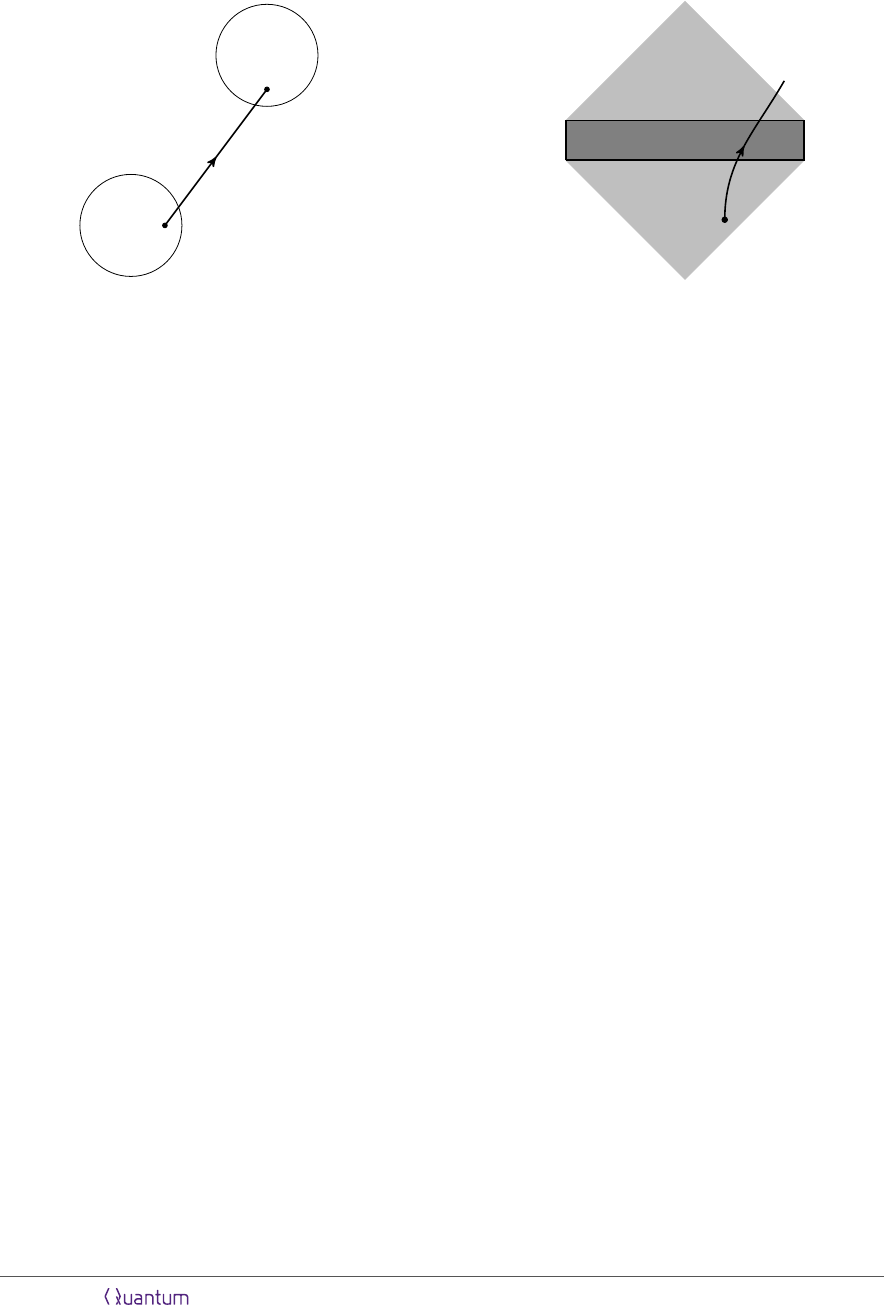Σ
i
Σ
j
q
i
q
j
(a)
Σ
p
(b)
Figure 2: Two geometric notions used in the text. a) Two causally connected regions. Two
spacetime regions Σ
i
and Σ
j
are said to be causally connected if there is a point q
i
in Σ
i
and q
j
in
Σ
j
such that there is a causal curve from q
i
to q
j
, or from q
j
to q
i
. b) The domain of dependence
(light grey) of a spacetime region Σ (dark grey). The domain of dependence is deﬁned as the set
of all points p in the spacetime such that all causal curves passing through p must also enter Σ.
Deﬁnition 4 The domain of dependence of a spacetime region Σ, denoted D(Σ),
is the set of all points p such that every causal curve through p must also enter Σ.
This deﬁnition is illustrated in ﬁgure 2b.
As a ﬁrst step towards the more general scenario consider the localization of a
quantum system to two authorized regions A
1
and A
2
.
Theorem 5 Given a quantum system initially localized near a spacetime point s, the
system may be localized to both of the spacetime regions A
1
and A
2
if and only if the
following two conditions hold.
i. A
1
and A
2
both have a point in the future light cone of s.
ii. A
1
and A
2
are causally connected.
Proof. First, note that if an authorized region is entirely outside the future light cone
of the start point then successfully localizing the system to that region would constitute
superluminal communication. Thus, the ﬁrst condition is necessary. To see necessity of
the second condition suppose there exists a protocol for localizing a quantum system to
two causally disjoint regions A
1
and A
2
. Then by deﬁnition it is possible to construct
the system by accessing the region A
1
, and by accessing A
2
. By causality however
accessing region A
1
cannot aﬀect the system constructed from A
2
, and vice versa, so it
would be possible to construct two copies of the quantum system. But this constitutes
cloning, so no such protocol can exist.
To understand suﬃciency we construct a task with the minimal properties speciﬁed
by the two assumed conditions. Such a task is shown in ﬁgure 3. There, a point p
1
A
1
Accepted in Quantum 2019-07-02, click title to verify 7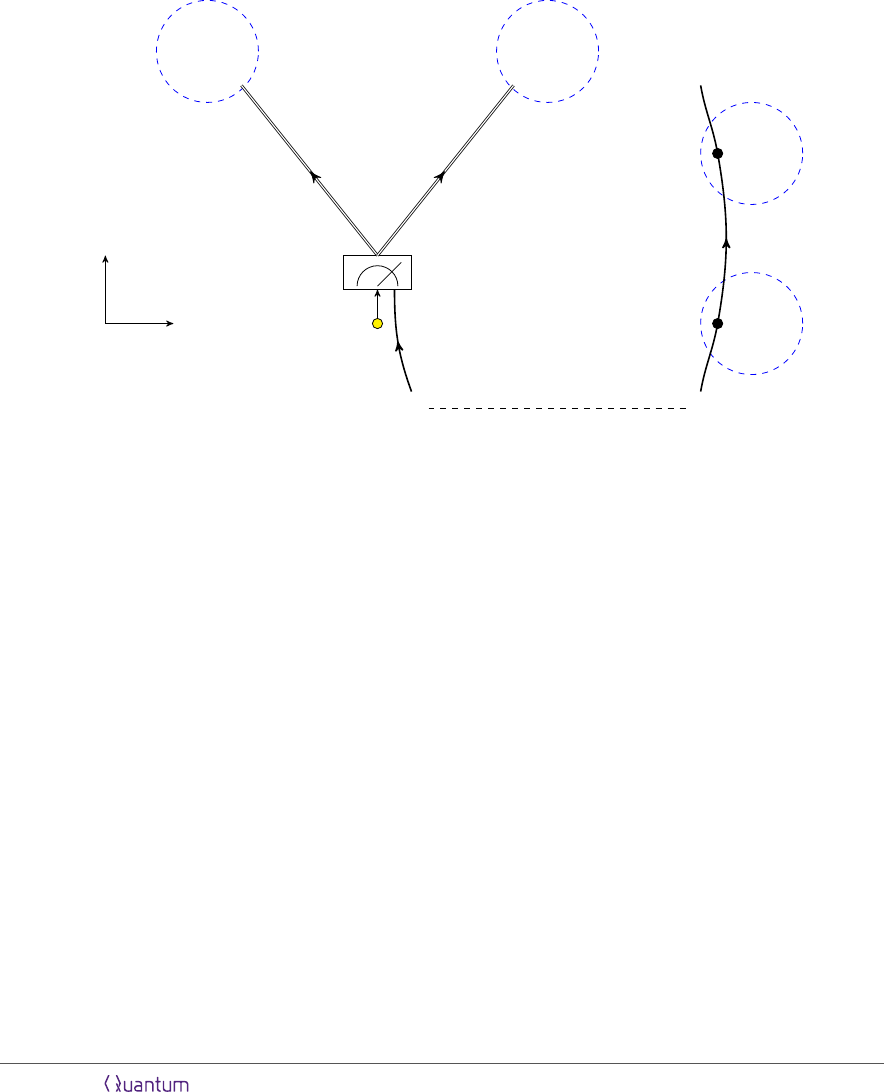A
1
A
2
sx
t
A
1
p
1
A
2
p
2
¯
E
E
|Ψ
+
i
Figure 3: An arrangement of two authorized regions that has the minimal requirements to satisfy
the conditions of theorem 5. By the ﬁrst condition A
1
and A
2
are causally connected. This
guarantees the existence of a point p
1
in A
1
which is in the causal future of some point p
2
in A
2
(up to relabelling). The second condition gives that each region have at least one point in the
future light cone of s. However, the regions A
1
and A
2
may be disconnected (as shown here) and
so satisfy this requirement while having the points p
1
, p
2
be outside the future light cone of s . To
localize a system A to both regions a maximally entangled state |Ψ
+
i
E
¯
E
is shared between s and
p
1
. Near to s the A system is teleported using this entanglement, and the entangled system at
p
1
is sent to p
2
. Meanwhile, the classical measurement outcomes from the teleportation protocol
are sent to the points in A
1
and A
2
which are in the causal future of s. Each region has both
the classical measurement outcomes and the entangled particle pass through it, so the A system
is localized to each.
Accepted in Quantum 2019-07-02, click title to verify 8is causally connected to p
2
A
2
, and each of A
1
and A
2
have a point in the future light
cone of s. However, p
1
and p
2
sit outside the future light cone of s. Nonetheless it is
straightforward to complete such a task. To do so a system E is maximally entangled
with
¯
E, then E is brought to s while
¯
E is brought to p
1
. At s, E is used to teleport the A
system onto the
¯
E system. The measurement outcome from the teleportation is sent to
A
1
and A
2
from s. Meanwhile,
¯
E is sent from p
1
to p
2
. Each authorized region contains
the classical measurement outcome and the system
¯
E, so accessing either region allows
reconstruction of A.
We can now move on to understanding localize tasks with arbitrary numbers of au-
thorized regions. We ﬁnd in particular that it is only the structure of causal connections
between pairs of regions and the start point that are needed to characterize a task as
possible or impossible.
Theorem 6 Given a quantum system A initially localized near a spacetime point s, the
system may be localized to each spacetime region A
i
in a collection {A
1
, ...., A
n
} if and
only if the following two conditions hold.
i. Each region A
i
has at least one point in the causal future of s
ii. Each pair of regions (A
i
, A
j
) is causally connected.
Proof. Necessity of the two conditions follows from the same arguments as in the two
region case given as theorem 5: localizing a system to a region outside of its future light
cone violates no signaling, and localizing a system to two spacelike separated regions
would allow two copies of the system to be produced.
To demonstrate suﬃciency we construct an explicit protocol for completing any task
satisfying the two conditions. To this end it is useful to introduce a directed graph G
which describes the causal structure of the task: for each authorized region A
i
introduce
a vertex, also labelled A
i
, to the graph. For each pair of regions (A
i
, A
j
) such that
there is a point in A
j
connected by a causal curve to a point in A
i
introduce a directed
edge (A
i
A
j
). An example of a task and its associated graph is given as ﬁgure 4.
From the no-cloning theorem it follows that some quantum information must be
shared between every pair of authorized regions. In our construction these quantum
systems that move between pairs of authorized regions form the shares of an error-
correcting code. In particular, for each edge in the graph G we associate one share. In
theorem 5 and ﬁgure 3 we showed how to localize a quantum system to two authorized
regions whenever they share a causal connection. We can execute this protocol on the
shares of our error-correcting code to ensure the share associated to edge A
i
A
j
is localized to both A
i
and A
j
. To complete the task then, our error-correcting code
should have the property that, given any vertex, the set of shares associated to the edges
attached to that vertex are suﬃcient to construct the initial system A. We illustrate
the requirement on this code in ﬁgure 5.
In fact, given that every pair of vertices in this graph share an edge, which is guar-
anteed by condition (ii), such error-correcting codes have already been constructed.
Accepted in Quantum 2019-07-02, click title to verify 9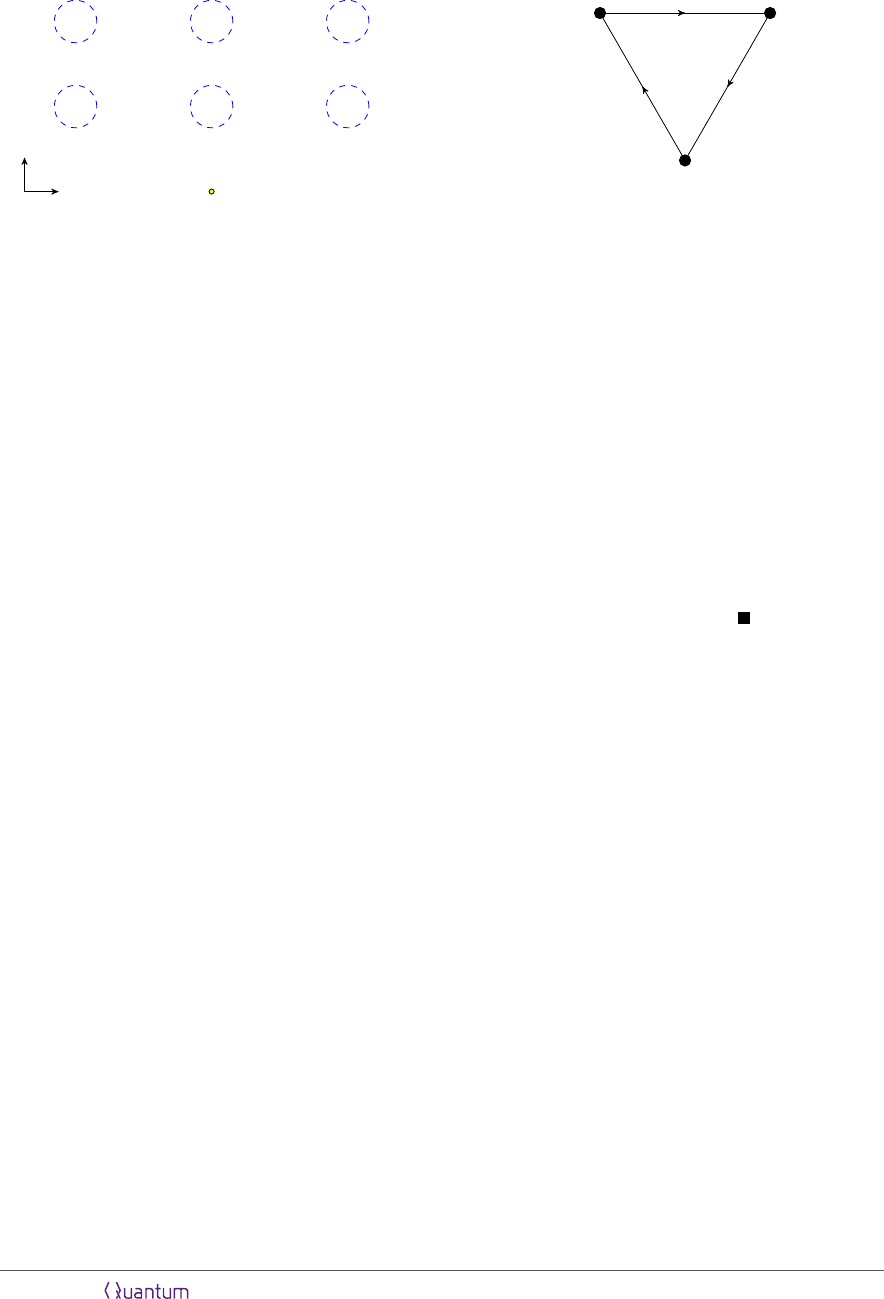A
3
A
1
A
1
A
2
A
2
A
3
x
t
s
(a)
A
1
A
2
A
3
(b)
Figure 4: An example of a task with three authorized regions A
1
, A
2
and A
3
. (a) The arrangement
of the regions in spacetime, notice that each region consists of two disconnected ball-shaped
regions. (b) The corresponding graph of causal connections, used in the proof of theorem 6 to
construct the error-correcting code needed to complete the task.
To encode ﬁnite-dimensional quantum systems we constructed such codes using the
codeword-stabilized formalism in the context of a similar summoning problem . Con-
structions for continuous variable systems have also been given  and then adapted
to the ﬁnite-dimensional case . In the code-word stabilized construction a single
logical qubit is recorded using 2 physical qubits for each edge in the graph, resulting in
a total of 2
n
2
physical qubits for n the number of authorized regions.
This result is particularly simple and expected from earlier work on summoning.
Indeed, the conditions for summoning to a collection of diamonds are the same as for
localizing to a collection of authorized regions (see , or appendix A).
2.2 Localizing and excluding quantum information
Now that we have an understanding of when and how a quantum system can be lo-
calized to many spacetime regions, we can approach the localize-exclude task. This
task includes a notion of unauthorized region, a region in spacetime from which the
system must be excluded in the sense described in the last section. Further, we will re-
quire that accessing an unauthorized region reveals no information about the quantum
system. We collect these ideas into the following deﬁnition.
Deﬁnition 7 A localize-exclude task involves two agencies, Alice and Bob, and is
speciﬁed by a tuple {A, s, {A
1
, ..., A
n
}, {U
1
, ..., U
m
}}, consisting of:
i. A quantum system A. In general A may be a subsystem of some overall pure state
|Ψi
AR
. The state on AR is unknown to both Alice and Bob.
ii. A start point s, at which Alice initially holds system A
iii. A collection of spacetime regions {A
1
, ..., A
n
}, which we call the authorized regions
Accepted in Quantum 2019-07-02, click title to verify 10A
1
A
2
A
3
A
4
(a)
A
1
A
2
A
3
A
4
(b)
Figure 5: Illustration of the functioning of the error-correcting code used in theorem 6. a) A
directed graph that describes the causal connections between the authorized regions of a localize
task. In this case the task involves four authorized regions. b) To complete the task, we employ an
error-correcting code that associates a share to each edge in the corresponding undirected graph.
The encoded qubit can be reconstructed from the shares associated with the edges attached to
any one vertex, corresponding to the sets of edges crossed by the purple arcs. For a single logical
qubit, the shares on each edge consist of two qubits. A detailed construction of the code can be
found in , and a more eﬃcient version in . For inﬁnite dimensional versions see .
iv. A collection of spacetime regions {U
1
, ..., U
m
}, which we call the unauthorized
regions
Bob will choose to access one of the A
i
or U
i
, and will attempt to construct the quantum
system A from his access. Alice successfully completes the task if both (a) Bob is able
to construct A when he accesses any one of the A
i
and (b) Bob learns no information
about A if he accesses any one of the U
i
.
If Alice successfully completes the localize-exclude task, we say she has localized system
A to the corresponding authorized regions while excluding it from the unauthorized
regions.
As an initial approach to understanding the localize-exclude task we can list oﬀ the
most basic restrictions that we expect to apply. First, the two restrictions occurring in
the context of the localize task are still relevant: the start point should have a point
from each authorized region in its future light cone, and there should be no causally
disjoint pairs of authorized regions. There are also additional restrictions relating to
the unauthorized regions however. In particular, we can never have an authorized
region A
i
be contained in the domain of dependence of an unauthorized region U
j
,
since then all information which enters A
i
also enters U
j
. Finally, the start point
too should not be contained in the domain of dependence of any unauthorized region.
We illustrate each these conditions in ﬁgure 6. Remarkably, a localize-exclude task
Accepted in Quantum 2019-07-02, click title to verify 11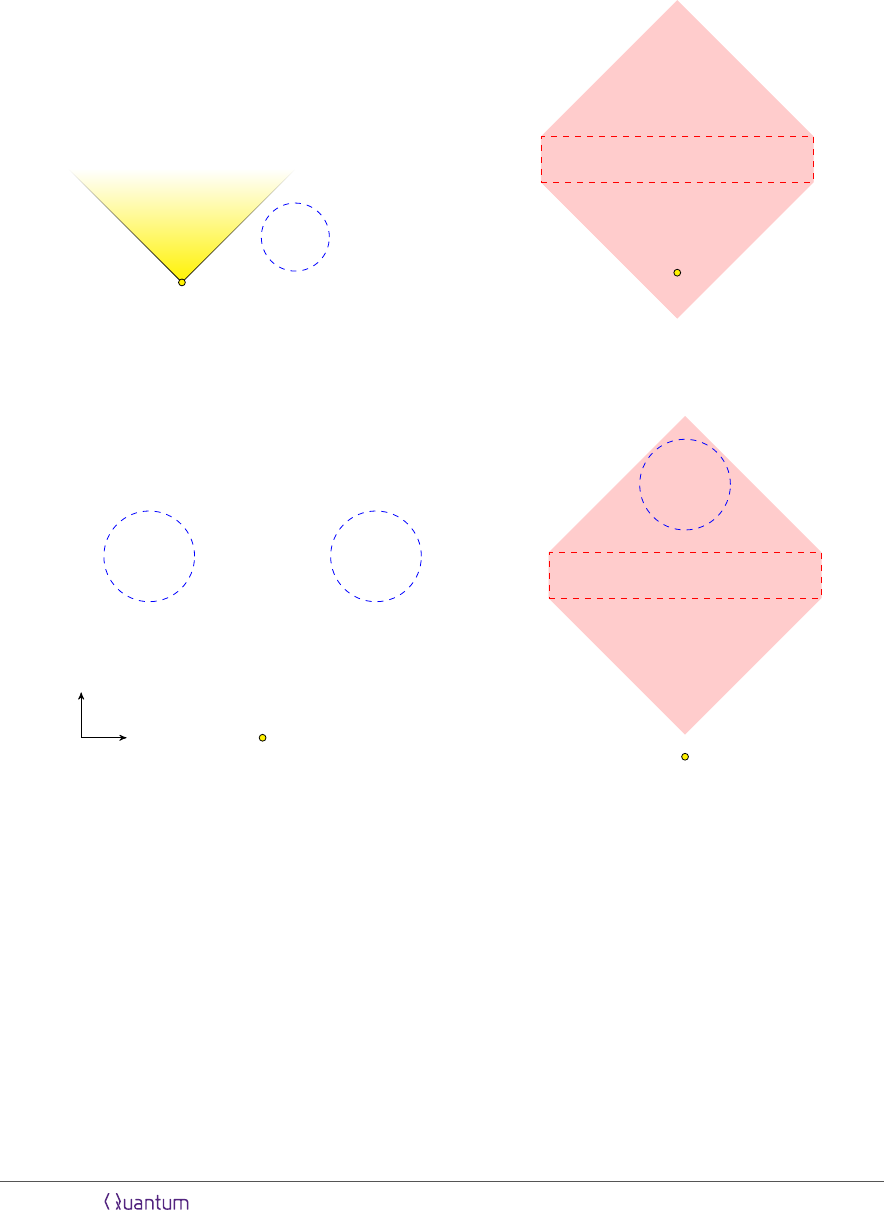s
A
1
(a)
s
U
1
(b)
A
1
A
2
sx
t
(c)
A
1
U
1
s
(d)
Figure 6: Four impossible localize-exclude tasks: (a) An authorized region is entirely outside the
future light cone of s, so system A can’t be localized there without violating the no-signalling
principle. (b) The initial location of the quantum system is in the domain of dependence of an
unauthorized region U
1
, so can be reconstructed from data in U
1
. (c) A quantum system cannot
be localized to both the spacetime regions A
1
and A
2
, due to the no-cloning theorem. (d) A
quantum system cannot be localized to A
1
without passing through the region U
1
, since there is
no causal curve which passes through A
1
and not U
1
. The red shaded region indicates the domain
of dependence of the unauthorized region U
1
. The yellow shading indicates the future light cone
of the start point.
Accepted in Quantum 2019-07-02, click title to verify 12{A, s, {A
1
, ..., A
n
}, {U
1
, ..., U
m
}} will turn out to be possible to complete so long as
none of the four situations in ﬁgure 6 occur.
As a warm-up to the general case, consider the example given in the introduction as
ﬁgure 1. There, a single unauthorized region blocks the path between two authorized
ones. As we illustrate in ﬁgure 7, it is nonetheless possible to complete the task using
the quantum one-time pad . Near the start point, a unitary U
k
is applied to A
with k chosen at random. The overall pure state is then U
k
I|Ψi
AR
. To an observer
who is unaware of the key k, the density matrix of the state is ρ
AR
=
P
k
1
|k|
(U
k
I)|ΨihΨ|(U
k
I). By carefully choosing the set of possible unitaries U
k
, one can arrange
that ρ
AR
= I
A
/d
A
ρ
R
, so that Bob has learned nothing about the A system whenever
he does not learn k. This is possible when A consists of n qubits and k consists of 4n
bits . Once encoded using the one-time pad, the A system is sent through both
authorized regions by allowing it to pass through the unauthorized region. An access
to the unauthorized region then only sees the maximally mixed state. The classical
key k is also sent to both authorized regions, but along trajectories that avoid the
unauthorized one.
A similar technique can be applied to the general case of many authorized and many
unauthorized regions. As we show in the proof of theorem 8 given below, the strategy is
to ﬁrst encode the A system into an error-correcting code so that it can be localized to
each authorized region. Then each share in that error-correcting code is encoded using
a classical string and the quantum one-time pad. We then leverage classical secret
sharing to allow us to get the encoding string to the needed authorized regions while
avoiding all the unauthorized regions.
We are now ready to state theorem 8 and give the proof. The proof of suﬃciency is
somewhat lengthy, so we have provided ﬁgure 8 which summarizes the key steps taken.
Theorem 8 Given a collection of authorized regions {A
1
, ..., A
n
}, unauthorized regions
{U
1
, ..., U
m
}, and start point s, a localize-exclude task is possible if and only if the
following three conditions are satisﬁed.
i. The starting location of the system A (a) has at least one point from each autho-
rized region in its causal future, and (b) is not in the domain of dependence of
any unauthorized region.
ii. Every pair of authorized regions (A
i
, A
j
) are causally connected.
iii. For every pair (A
i
, U
j
) of authorized and unauthorized regions, A
i
is not contained
in the domain of dependence of U
j
.
Proof. The necessity of conditions (i)(a) and (ii) follow from the same arguments as
in theorem 6. To argue the necessity of condition (iii), notice that if A
i
is contained
in the domain of dependence of U
j
, then the state of the quantum ﬁelds within A
j
is determined by unitary evolution from the ﬁelds within U
i
. Then whenever the A
system can be determined from A
i
it is also possible to recover it from U
j
. Condition
(i)(b) is necessary for the same reason.
Accepted in Quantum 2019-07-02, click title to verify 13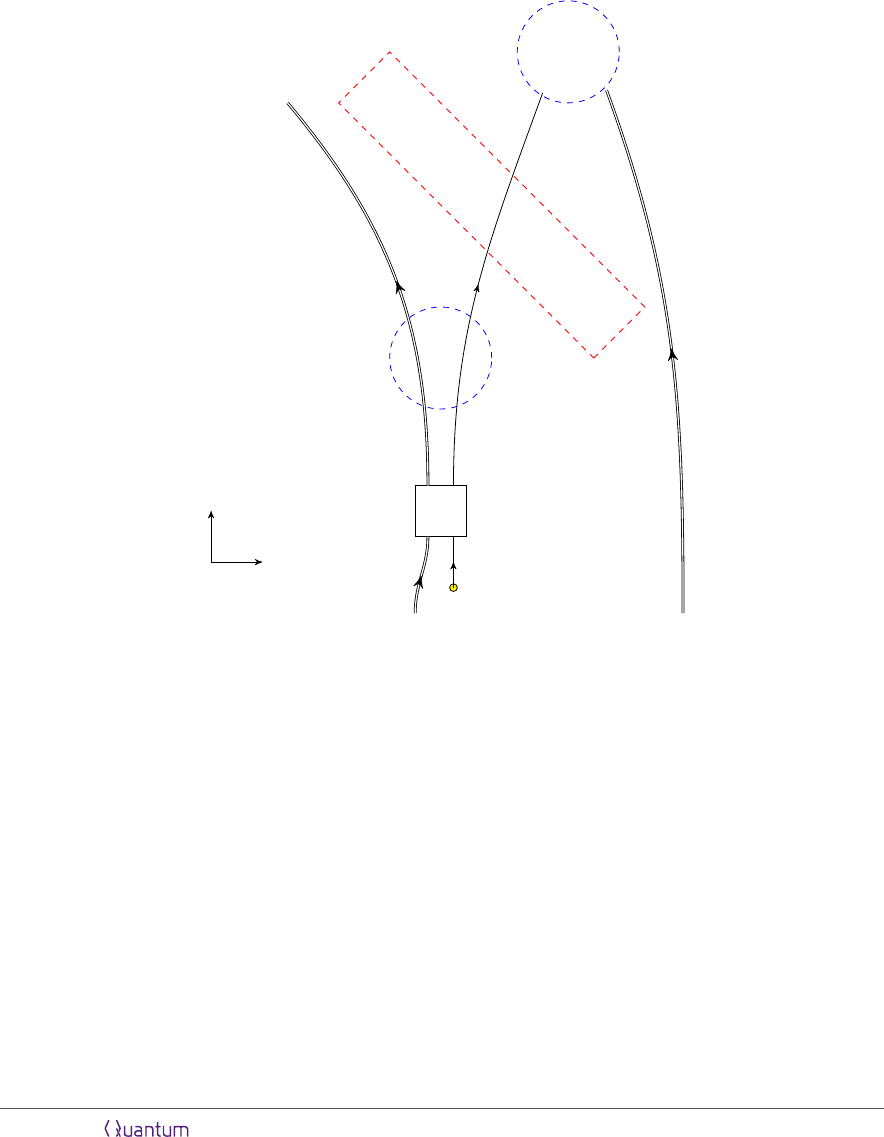A
2
A
1
A
U
x
t
U
k
kk
Figure 7: Illustration of the protocol for completing a localize-exclude task with two authorized
regions and one unauthorized region that satisfy the conditions of theorem 8. In the distant past,
Alice prepares copies of the classical string k. She brings one copy of k to each of A
1
and A
2
along
a path which does not cross U this is always possible by condition (iii). She must also bring
the classical string to the start point s, and encode the A system using the quantum one-time
pad . The overall state on A and its purifying system R is then of the form (U
k
I)|Ψi
AR
.
The encoded system A is sent through both authorized regions. By following this protocol both
authorized regions contain k and the encoded A system, while the unauthorized region contains
the encoded system only.
Accepted in Quantum 2019-07-02, click title to verify 14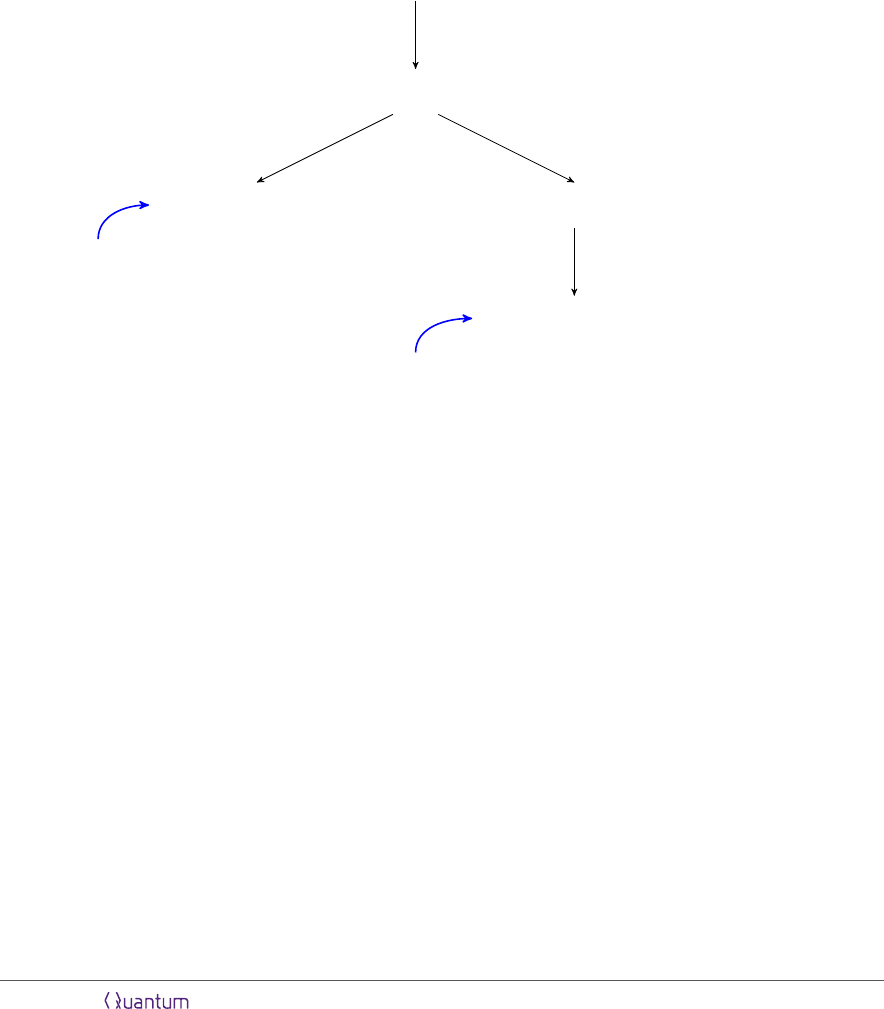(A, s, {A
1
, ..., A
n
}, {U
1
, ..., U
m
})
×1
(S
ij
, s, {A
i
, A
j
}, {U
1
, ..., U
m
})
×
(
n
2
)
(S
ij
, s, {A
i
, A
j
}, )
×1
Teleportation
(k
ij
, −∞, R
i
, {U
1
, ..., U
m
})
×3
(k
l
ij
, −∞, R
i
, U
l
)
×m
Send string on causal
curve through R
i
\ U
l
Theorem 6
((m, m)) classical
secret sharing scheme
Figure 8: Diagram of the suﬃciency proof of theorem 8. In three steps, the proof reduces
completing the localization task on the system A with n authorized sets and m unauthorized sets,
denoted by (A, s, {A
1
, ..., A
n
}, {U
1
, ..., U
m
}), to completing
n
2
instances of (S
ij
, s, {A
i
, A
j
}, )
on quantum shares, and 3m
n
2
instances of (k
l
ij
, −∞, R
i
, U
l
) on classical shares, where the region
R
i
may be either the start point or an authorized region. The notation −∞ indicates the share is
available at early times. The ﬁrst step in the protocol is to recycle the error-correcting code from
theorem 6 to encode the A system into shares S
ij
. At the second step, the one-time pad is applied
to each of the S
ij
. This allows the unauthorized regions to be avoided by introducing additional
classical shares, but without the need for further uses of quantum error-correcting codes.
Accepted in Quantum 2019-07-02, click title to verify 15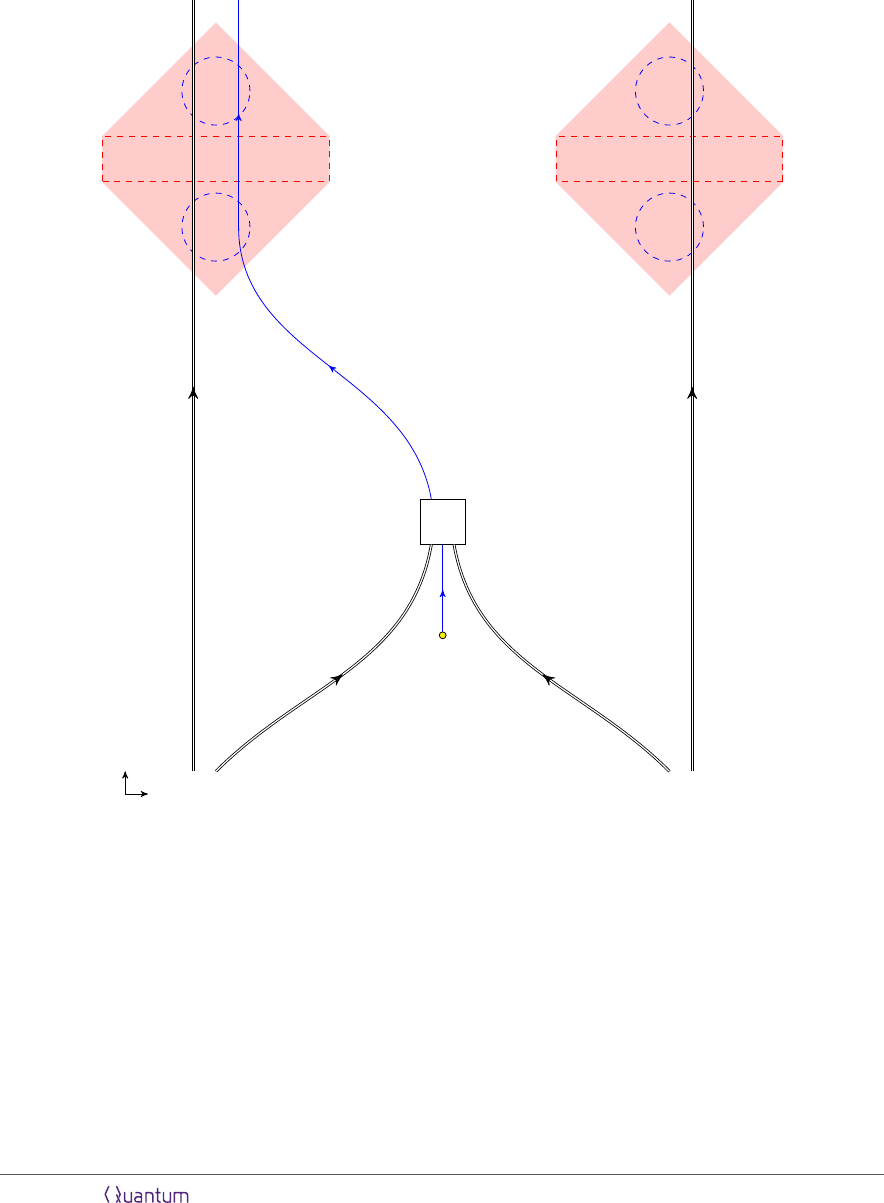U
1
U
2
A
1
A
2
A
2
A
1
A
x
t
U
k
k
2
k
1
Figure 9: An example of a localize-exclude task and illustration of the protocol provided by theorem
8 for its completion. Near the start point the system A is encoded using the quantum one-time
pad and sent (along the blue curve) through both authorized regions. The string k satisﬁes
k = k
1
k
2
, so that k
1
, k
2
form the two shares of a ((2, 2)) secret sharing scheme. k
1
is sent
through A
1
and A
2
while avoiding U
1
, while k
2
is sent through A
1
and A
2
while avoiding U
2
.
Consequently, each A
i
contains all of the classical shares k
i
along with the encoded A system,
while each U
i
is missing one k
i
.
Accepted in Quantum 2019-07-02, click title to verify 16To demonstrate suﬃciency we construct an explicit protocol to complete the task
in the case where all three conditions are true. It is useful to recall the notation
(A, s, {A
1
, ..., A
n
}, {U
1
, ..., U
m
}), which describes a localize-exclude task by specifying
the system on which we must complete the task, the start point, authorized regions, and
unauthorized regions. As a ﬁrst step in constructing our protocol, we encode the system
A into the error-correcting code used in theorem 6. Using this code and localizing each
share in the code to its two associated authorized regions would localize the system
to each authorized region. However, here we also need to exclude the system from all
of the unauthorized regions. To do this, we will localize each share S
ij
to A
i
and A
j
while also avoiding every unauthorized region. In other words, encoding A into the
codeword stabilized code reduces completing the original task to completing the tasks
(S
ij
, s, {A
i
, A
j
}, {U
1
, ..., U
m
}) for every share S
ij
.
By using the quantum one-time pad and classical secret sharing it is possible to
further reduce completing the (S
ij
, s, {A
i
, A
j
}, {U
1
, ..., U
m
}) task. In particular, at s
use the quantum one-time pad to encode the share S
ij
using some classical string k
ij
. We
may freely send the encoded share through A
i
and A
j
so long as the classical string k
ij
is
kept out of all of the unauthorized regions, and is made available at s, A
i
, and A
j
. Thus,
ij
, s, {A
i
, A
j
}, {U
1
, ..., U
m
}) is equivalent to completing (S
ij
, s, {A
i
, A
j
}, )
along with (k
ij
, −∞, {s, A
i
, A
j
}, {U
1
, ..., U
m
})
3
.
To ﬁnish the protocol, we ﬁrst notice that theorem 6 shows that we can complete
any task of the form (S
ij
, s, {A
i
, A
j
}, ) given that conditions (i)(a) and (ii) hold. The
ij
, −∞, {s, A
i
, A
j
}, {U
1
, ..., U
m
}) is also easily handled. Note that since the task
is to be completed on a classical string, we can produce three copies of k
ij
and worry
separately about sending the string to s and each of A
i
and A
j
, so we have to complete
three instances of (k
ij
, −∞, R, {U
1
, ..., U
m
}), where R can be s, A
i
or A
j
. To complete
these, encode k
ij
into an ((m, m)) secret sharing scheme
4
with shares k
l
ij
. Then complete
l
ij
, −∞, R, U
l
). This completes the task with all m unauthorized regions
since the classical string is kept out of U
l
so long as at least one of the shares in the
((m, m)) scheme is.
It remains to complete the tasks of the form (k
l
ij
, −∞, R, U
l
). When R is one of the
authorized sets, condition (iii) guarantees that R is not in the domain of dependence
of U
l
, which means there is a causal curve passing through R which does not enter U
l
.
To complete the task, simply send k
l
ij
along this curve. When R is the start point s,
condition (i)(b) guarantees there is a causal curve passing through s and not U
l
, so
again we can complete this task.
An example of the protocol used in this proof is given as ﬁgure 9.
3
We’ve introduced the notation −∞ to indicate the start point is located in the distant past. This
is the appropriate task to consider completing on the classical system k
ij
as Alice may prepare these
strings at some early time.
4
A ((k, n)) secret sharing scheme is one where any k of the n total shares can be used to reconstruct
the secret while any k 1 shares reveal nothing about the secret. A ((m, m)) scheme is the appropriate
one here because we want every share to be needed to reconstruct k
ij
.
Accepted in Quantum 2019-07-02, click title to verify 17Σ
1
Σ
2
Σ
3
s
Figure 10: Example of the embedding of a secret sharing scheme with arbitrary access structure
into a localize-exclude task. We consider a secret sharing scheme that involves three parties,
and has authorized sets S
1
= {1, 2}, S
2
= {2, 3} and S
3
= {1, 2, 3}, with all other subsets of
parties deemed unauthorized. In the corresponding localize-exclude task, the three parties become
three causally disjoint spacetime regions Σ
1
, Σ
2
and Σ
3
. Further, this localize-exclude task has
authorized regions A
1
= Σ
1
Σ
2
, A
2
= Σ
2
Σ
3
and A
3
= Σ
1
Σ
2
Σ
3
. The start point s has
been placed at an early enough time that all the Σ
i
are in its future light cone.
Earlier we mentioned the similarity of conditions (ii) and (iii) to corresponding con-
ditions for quantum secret sharing. A quantum secret sharing scheme  is speciﬁed
by an access structure, with the access structure consisting of subsets of parties deemed
authorized and subsets deemed unauthorized. A quantum secret sharing scheme can
be constructed under two conditions : (a) (no-cloning) no two authorized sets can
be disjoint and (b) (monotonicity) no authorized set can be contained within an unau-
thorized set. Conditions (ii) and (iii) of the localize-exclude theorem are exactly these
conditions rephrased in a context appropriate to spacetime.
Beyond this similarity, we can embed any secret sharing scheme into a localize-
exclude task. Consider n parties, Bob
1
,...,Bob
n
, who each can potentially access an
associated spacetime region Σ
i
. Take the authorized and unauthorized regions to consist
of unions of Σ
i
’s so that a full authorized region A
i
can be accessed only if some
collection of Bobs agree to cooperate. Choose the regions Σ
i
to be all causally disjoint.
In this setting two authorized regions being causally connected occurs if and only if
they share a Σ
i
. Then condition (ii) of theorem 8, which requires causal connections
between authorized regions, reduces to the requirement that every pair of authorized
regions share at least one Σ
i
. This is exactly the no-cloning requirement on secret
sharing. Further, condition (iii) reduces to no U
i
= Σ
i1
... Σ
in
containing as a
subset some A
j
= Σ
j1
... Σ
j2
under the same restriction of having causally disjoint
Σ
i
. This is just the monotonicity condition on quantum secret sharing schemes. Finally,
to embed our quantum secret sharing task into a localize-exclude task we should ensure
Accepted in Quantum 2019-07-02, click title to verify 18that condition (i) becomes trivial, which we can do by sending the start point s to an
early time. We illustrate the embedding of a secret sharing task into a localize-exclude
Theorem 8 shows that completing a localize-exclude task with unauthorized regions
requires only the same quantum error-correcting code as used in the case with no unau-
thorized regions. Hiding the system from the unauthorized regions can be accomplished
using only the quantum one-time pad and classical secret sharing. This is similar to
the approach taken in , where quantum error-correcting codes are combined with
the quantum one-time pad to yield quantum secret sharing schemes. By using the eﬃ-
cient error-correcting code underlying our protocol however, we arrive at a particularly
eﬃcient construction of quantum secret sharing schemes. In particular we ﬁnd that
there is a universal quantum error-correcting code with 2
n
2
shares for n the number
of authorized sets which, along with uses of the one-time pad and classical secret shar-
ing, constructs quantum secret sharing schemes with arbitrary access structures. Using
Shamir’s method  to construct the classical secret sharing schemes, the 3m
n
2
in-
stances of the ((m, m)) classical scheme will each require O(m log m) bits, where m was
the number of unauthorized sets. In total, O(n
2
) qubits and O(m
2
n
2
log m) classical
bits are used in the localize-exclude construction. This provides the ﬁrst construction
of quantum secret sharing schemes using a number of qubits polynomial in the num-
ber of authorized sets. Previously, eﬃcient constructions were known for threshold
schemes and certain other special access structures. (See, e.g. [26, 27, 24].) Since the
number of unauthorized sets can grow exponentially with n, the classical bits used can
be exponentially large. This is to be expected since it is conjectured to be impossi-
ble to construct classical secret sharing schemes for arbitrary access structures without
consuming exponential resources .
3 State-assembly
3.1 State-assembly with authorized regions
In the localize-exclude task Bob can access any one of a set of spacetime regions. Alice,
who holds various quantum systems within those regions, is helpless to prevent Bob’s
access. In an alternative scenario we can have Bob request information from Alice.
Alice is free to comply with the request or to reject it, and hand over no information.
Certain sets of requests are deemed authorized, others unauthorized. Sets of requests
corresponding to authorized sets should result in Alice handing over suﬃcient informa-
tion for the system to be reconstructed; requests to unauthorized sets should reveal no
information about the system. Considering such scenario’s leads us to construct the
Before giving a precise deﬁnition of the task we introduce a few constructions. To
specify locations where Bob may request the system we designate certain spacetime
points as call points c
i
. At each call point a bit b
i
{0, 1} is revealed to Alice. To
Accepted in Quantum 2019-07-02, click title to verify 19each call point there corresponds a return point r
i
. Together, a call point and the
corresponding reveal point deﬁne a causal diamond.
Deﬁnition 9 The causal diamond D
i
is deﬁned as the intersection of the points in
the past light cone of r
i
with those in the future light cone of c
i
.
If b
i
= 1 we say the diamond D
i
has been called to. The causal diamond represents
the spacetime region in which it is possible to both know that a call was received, and
to use this information to inﬂuence what is handed over at the corresponding return
point.
We can now deﬁne the state-assembly task.
Deﬁnition 10 A state-assembly task involves two agencies, Alice and Bob, and is
speciﬁed by a tuple {A, s, {A
1
, ..., A
n
}, {U
1
, ..., U
m
}}, consisting of
i. A quantum system A. In general A may be a subsystem of some overall pure state
|Ψi
AR
. The state on AR is known to Alice and unknown to Bob.
ii. A start point s at which Alice initially holds A.
iii. A collection of authorized sets of diamonds {A
1
, ..., A
n
}. Each authorized set
consists of a collection of diamonds, A
i
= {D
1i
, ..., D
ki
}.
iv. A collection of unauthorized sets of diamonds {U
1
, ..., U
m
}. Each authorized set
consists of a collection of diamonds, U
i
= {D
1i
, ..., D
ki
}.
Alice will receive calls at a subset of the D
i
. If the set of called to diamonds corresponds
to an authorized set, Alice should return quantum systems and classical instructions
suﬃcient to reconstruct A at the associated reveal points r
i
. If the set of calls corre-
sponds to an unauthorized set, the systems she hands over should reveal no information
about A. Further, no set of calls should result in Alice returning systems suﬃcient to
construct two copies of the system.
There are a few points to clarify regarding this deﬁnition. First, Alice need not hand
the system over at any one of the called to diamonds. Instead the systems she hands
over at the called to diamonds should together be suﬃcient to recover the A system.
Second, calls to sets of diamonds not speciﬁed as authorized or unauthorized may result
in the system being handed over Alice still completes the task successfully so long
as she does not hand over two copies of the system.
In state-assembly Alice knows the state |Ψi
AR
and can potentially prepare many
copies of the A system. This diﬀers from the localize-exclude task and earlier work on
summoning. However, we have also required that she never hand over more than one
copy of A. As discussed in more detail in appendix A, this is actually leads to conditions
on state-assembly that are equivalent to having Alice hold an unknown state. We have
chosen to discuss this task from the perspective of a known quantum state however as
it is more natural in the context of the application given in section 4.
Accepted in Quantum 2019-07-02, click title to verify 20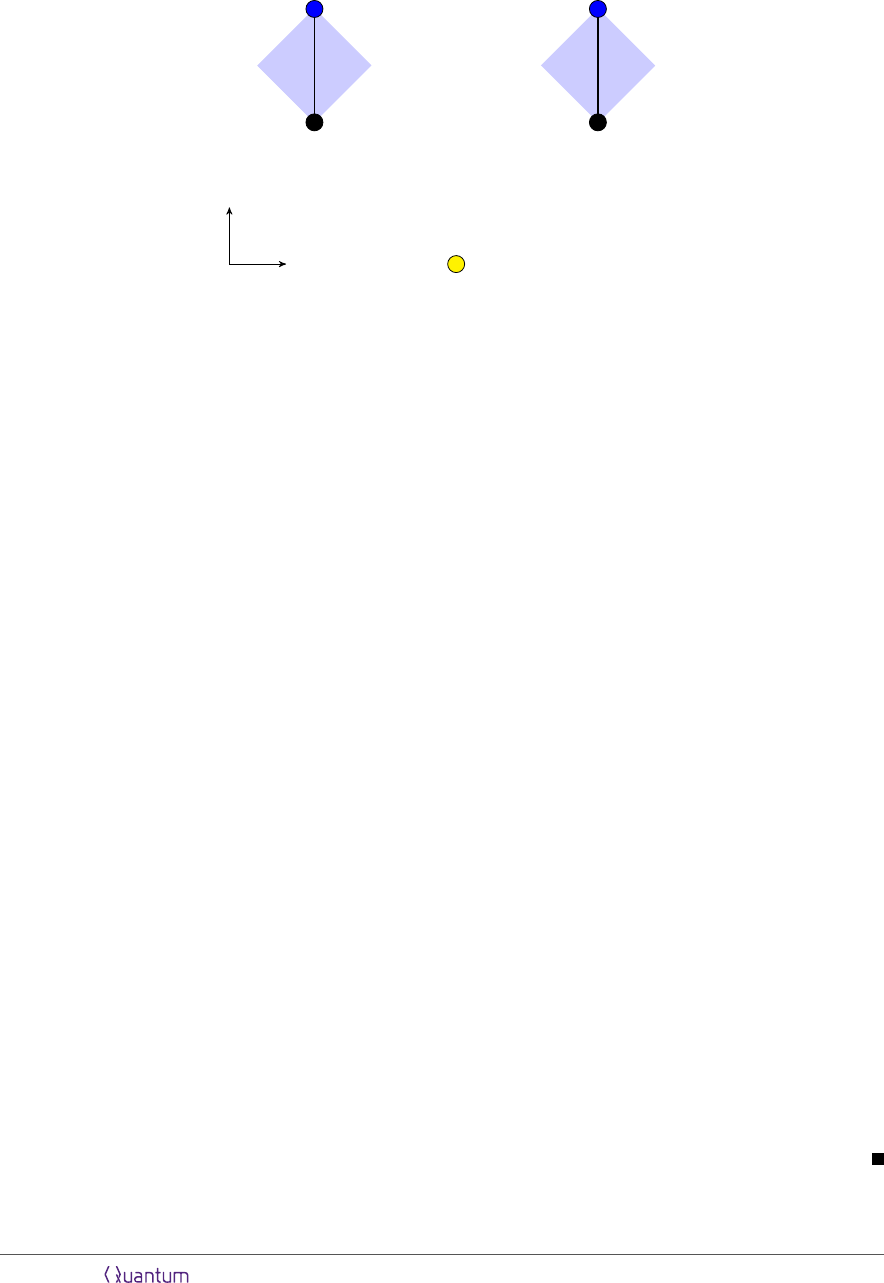s
c
1
c
2
r
1
r
2
x
t
Figure 11: A state-assembly task with two call-return pairs. A call to c
1
is required to result in the
system returned at r
1
, and likewise for c
2
and r
2
(indicated by the black lines), while a call to both
shouldn’t result in more than one copy of the system being turned over. This task is impossible
as shown by theorem 11, because r
2
is outside the future light cone of c
1
and r
1
is outside the
future light cone of c
2
. In the language of deﬁnitions 9 and 10, c
1
and r
1
form a causal diamond
D
1
(shown in blue), and the authorized set A
1
consists of the single diamond D
1
(similarly for c
2
and r
2
).
Before discussing more general constructions we begin with the simplest state-
assembly task, illustrated in ﬁgure 11, and prove a no-assembly theorem. In this scenario
there are just two authorized sets A
1
and A
2
.
Theorem 11 Consider a state-assembly task with authorized sets A
1
and A
2
which are
causally disconnected. Then this assembly task is impossible to complete with a perfect
success rate.
Proof. For Alice to successfully complete the assembly task, she must have a protocol
which
i. Returns suﬃcient information to construct the system when A
1
or A
2
calls.
ii. Hand over information suﬃcient to construct at most one copy of the system for
any set of calls.
We can straightforwardly show that any protocol which satisﬁes the ﬁrst requirement
cannot satisfy the second, and consequently there is no such successful protocol. Indeed,
suppose both A
1
and A
2
1
and A
2
are causally disjoint Alice’s
agents at the diamonds in A
1
cannot distinguish this situation from one where only
A
1
has been called to. By (i) then they hand in suﬃcient information to construct
the system. Similarly, Alice’s agents at A
2
will also hand in suﬃcient information to
construct the system. Since Bob may now construct two copies of A, (ii) is violated.
We see that completing the assembly task to causally separated regions is impossible.
Notice that it is essential that the Bobs may give calls to both diamonds: the possibility
Accepted in Quantum 2019-07-02, click title to verify 21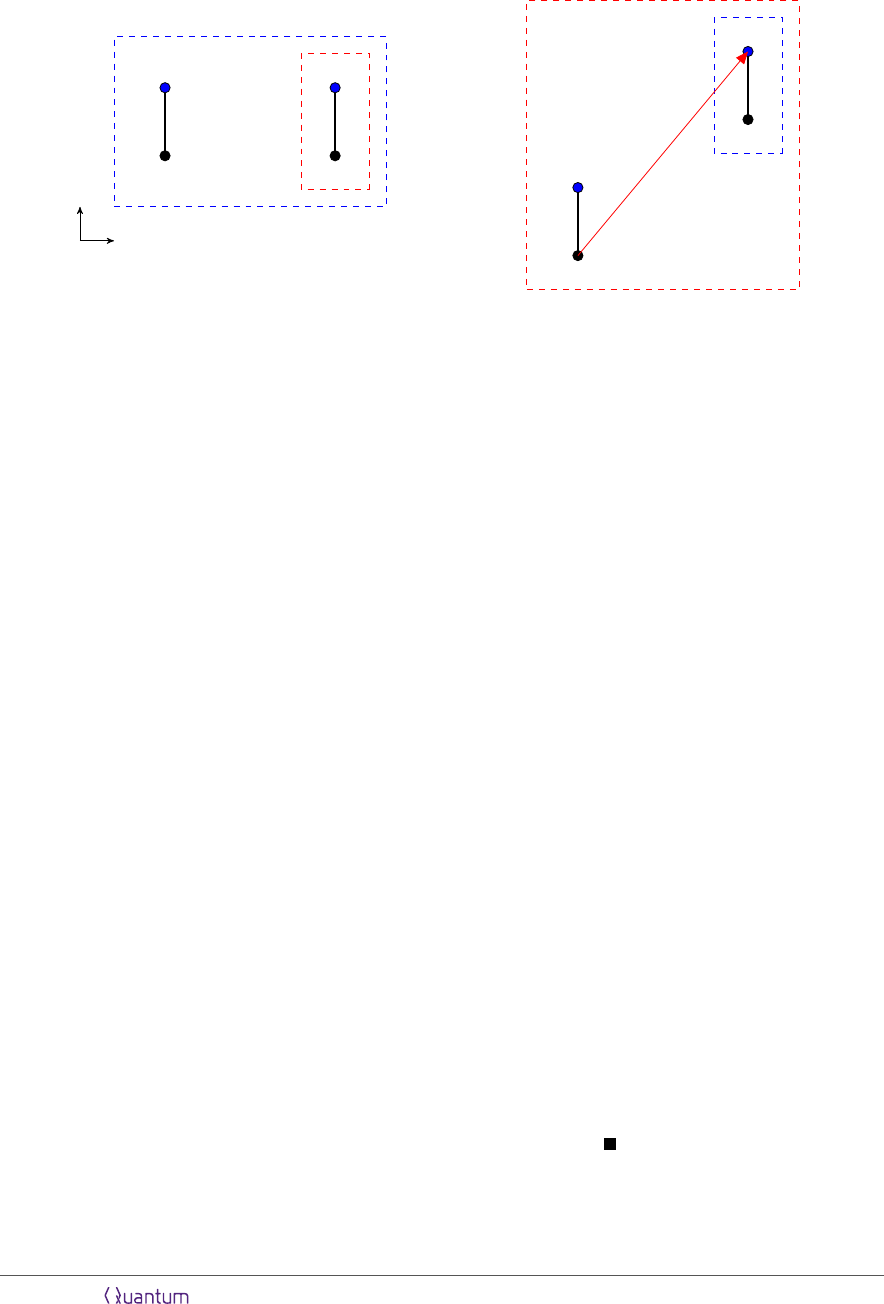c
1
c
2
r
1
r
2
x
t
(a)
c
1
c
2
r
1
r
2
(b)
Figure 12: Illustration of condition (iii) in theorem 13. Dashed red boxes enclose unauthorized sets
while dashed blue boxes enclose authorized sets. The condition states that every pairing (A
a
, U
i
)
of authorized with unauthorized set must have either (a) A
a
\ U
i
6= or (b) U
i
\ A
a
is causally
connected to A
a
.
of a call to A
1
A
2
along with the requirement that Alice allow assembly of not more
than one copy of the system leads to Alice being unable to complete the task successfully.
Next, we look at a wider class of assembly tasks involving an arbitrary number of
authorized sets {A
i
}.
Theorem 12 An assembly task with authorized sets {A
1
, ..., A
n
} and start point s can
be completed with a perfect success rate if and only if the following conditions hold.
i. The return point of at least one diamond from each authorized set is in the causal
future of the start point.
ii. Every pair of authorized sets (A
i
, A
j
) are causally connected.
Proof. The ﬁrst condition is necessary by no-signalling. The necessity of the second
condition follows from the same argument as given in theorem 11.
We can use theorem 8 to show suﬃciency of these conditions. There, we constructed
an explicit protocol that localizes the system to each authorized region. In particular,
the system is recorded as classical teleportation data and shares in a quantum error-
correcting code. To complete the assembly task then, Alice should execute the local-
ization protocol from theorem 8, with the authorized sets of diamonds considered as
authorized regions. Then to complete the assembly task Alice need only hand over the
classical and quantum data in A
i
Notice that this protocol automatically ensures Bob cannot give calls to receive two
copies of the system, since Alice only uses one copy of A.
Accepted in Quantum 2019-07-02, click title to verify 223.2 State-assembly with authorized and unauthorized regions
We can now proceed to characterize the state-assembly tasks with both authorized
and unauthorized sets that can be completed by Alice. The diﬃculty here for Alice is
diﬀerent than in the case of localize-exclude. In localize-exclude, she had to keep the
system out of a region U
i
i
. Now, Alice’s
labs are secure. However the sets of spacetime points corresponding to an authorized
call can be overlapping with those corresponding to an unauthorized call. This means
that locally she may not be able to tell an authorized and unauthorized call apart.
To understand under what conditions Alice can avoid an accidental reveal of the
system to an unauthorized set of diamonds, we can ﬁrst consider a task of the form
(A, s, A , U ) having one authorized and one unauthorized set of diamonds. In this case,
Alice can be successful if either (a) there is a diamond in A which is not in U , since
then she can turn over the system at that diamond only when there is a call there or
(b) there is a diamond D
in A which, although it is in U , is positioned such that
Alice can tell at D
whether the global set of calls is authorized or unauthorized. In
particular, this occurs exactly when there is a diamond in U \ A which is causally
connected to A . Figure 12 illustrates these two possibilities.
We now state and prove the theorem characterizing the state-assembly tasks with
authorized and unauthorized sets of diamonds.
Theorem 13 A state-assembly task with authorized sets {A
a
} and unauthorized sets
{U
i
} and start point s can be completed if and only if the following three conditions
hold:
i. The return point of at least one diamond from each authorized set is in the causal
future of the start point.
ii. Each pair of authorized sets (A
a
, A
b
) is causally connected.
iii. Each pair (A
a
, U
i
) of authorized with unauthorized sets has the property that either
A
a
\ U
i
6= or U
i
\ A
a
is causally connected to A
a
.
Proof. The necessity of conditions (i) and (ii) follow from the same arguments as in
theorem 12. To see the necessity of condition (iii), consider that its negation is that
both A
a
U
i
and U
i
\A
a
is not causally connected to A
a
. Then Alice’s agents in the
diamonds of A
a
, should they receive calls, cannot distinguish a call to A
a
from a call to
U
i
since they are causally disconnected from diamonds in U
i
\A
a
. In order to complete
the task, Alice must always hand the system over to A
a
when she receives a call there.
She will then also always hand over the system when the call is to U
i
To demonstrate suﬃciency we construct an explicit protocol to complete the task
in the case where all three conditions are true. Using the error-correcting code con-
structed from the graph of causal connections (also used in theorems 6, 8, and 12) we
can reduce the initial (A, s, {A
1
, ..., A
n
}, {U
1
, ..., U
m
Accepted in Quantum 2019-07-02, click title to verify 23(S
ij
, s, {A
i
, A
j
}, {U
1
, ..., U
m
}), where the S
ij
are the shares of the error-correcting code
associated to the i j pair of regions.
To complete the (S
ij
, s, {A
i
, A
j
}, {U
1
, ..., U
m
}) tasks, we encode the share S
ij
using
the quantum one-time pad with some classical randomness k
ij
. Now notice that we can
complete the (S
ij
, s, {A
i
, A
j
}, {U
1
, ..., U
m
ij
, s, {A
i
, A
j
}, ) on
the quantum share S
ij
and (k
ij
, s, A
i
, {U
1
, ..., U
m
}) and
(k
ij
, s, A
j
, {U
1
, ..., U
m
}) on the classical string. Stated another way, the use of the one-
time pad lets us ignore avoiding the unauthorized sets when considering the quantum
data, and only worry about not handing the classical string over at the unauthorized
sets.
To complete the tasks of the form (k
ij
, s, A